Get Started »

# Data Plots & Best-Fit Curves

Use ListPlot to visualize data as a scatterplot:

 In:=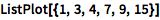⨯ `ListPlot[{1, 3, 4, 7, 9, 15}]`
 Out=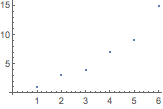Or display information as a chart:

 In:=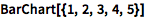⨯ `BarChart[{1, 2, 3, 4, 5}]`
 Out=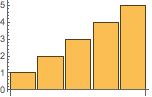There are also specialized functions for time series, financial data and much more.

Multiple datasets are automatically colored differently:

 In:=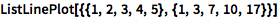⨯ `ListLinePlot[{{1, 2, 3, 4, 5}, {1, 3, 7, 10, 17}}]`
 Out=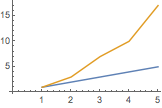You can change the style and appearance of plots using options like PlotTheme.

Find a curve of best fit with the Fit command:

({1,x,x2} means a quadratic fit over x.)
 In:=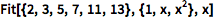⨯ `Fit[{2, 3, 5, 7, 11, 13}, {1, x, x^2}, x]`
 Out=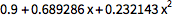Use Show to compare the curve with its data points:

 In:=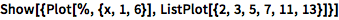⨯ `Show[{Plot[%, {x, 1, 6}], ListPlot[{2, 3, 5, 7, 11, 13}]}]`
 Out=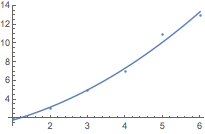QUICK REFERENCE: Data Visualization `»`

QUICK REFERENCE: Curve Fitting & Approximate Functions `»`